### Step 1: determining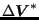In this step the first correction to the momentum is determined. To this end the Right Hand Side (RHS) of the equation system is calculated (this is done in mafillv1rhs.f and e_c3d_v1rhs.f). Notice that at this point the RHS is calculated not only theequation system, but also for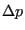(partially),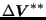(partially),,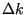and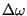(incompressible fluids) and for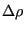(partially),,,and(compressible fluids). This saves time since any auxiliary variables have to calculated only once. Furthermore, this part is parallelized (multithreading) since it involves a loop over all elements which can be nicely cut into pieces. The equations are solved in routine solveeq in an iterative way (not only forbut also for,and, which are used later on in the algorithm). This is necessary, since the LHS has been approximated by lumping. The number of iterations is set in solveeq.f and is called maxit. Right now, it has the value 1, which means that no iterations take place. If the user wishes to change this, the source code has to be recompiled. The solution of the equations is stored in field v(1..nk,1),v(1..nk,2) and v(1..nk,3)). Notice thatis not added to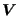at this point. Next,is changed such that the velocity boundary conditions are matched. These conditions are applied in the form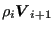, where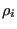is the density at the start of the increment and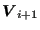is the velocity boundary condition corresponding to the time at the end of the increment (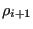is not known at this point).